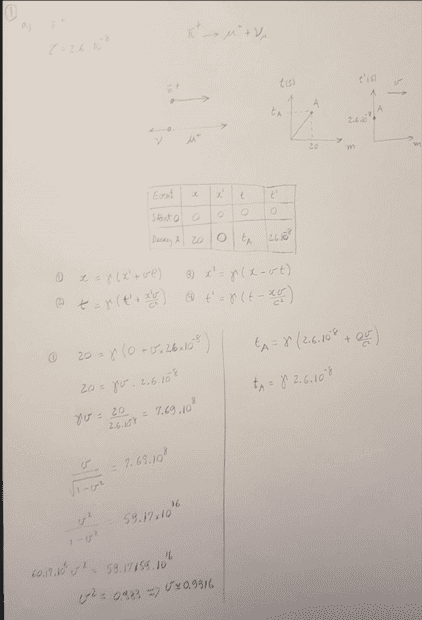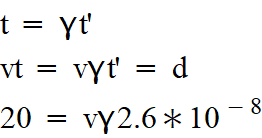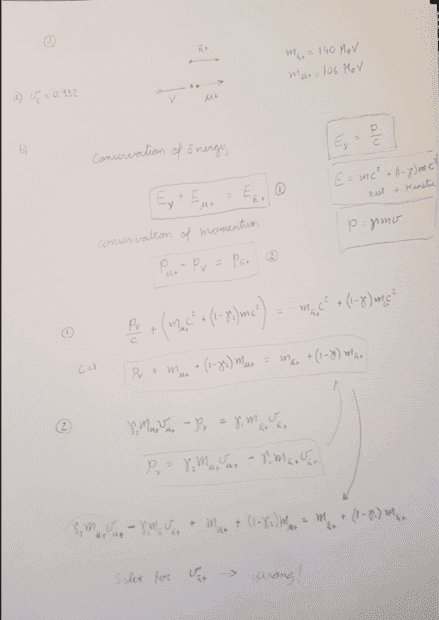# Lorentz Transformations

• R3ap3r42

#### R3ap3r42

Homework Statement
Another exercise on Lorentz - particle decay
Relevant Equations
Lorentz transformations, conservation of momentum and energy
A π+ meson is an elementary particle with a mean lifetime, defined in its rest frame, of τ = 2.60×10−8 s. The meson decays to a muon (µ+) and a neutrino (νµ) via the reaction π+ → µ+ + νµ. A π+ traveling in the laboratory decays so that the µ+ travels in the same direction as the original π+ and the νµ travels in the opposite direction.

a) At what speed must a typical π+ be traveling if it is to move a distance of 20 m in the laboratory from the point where it is produced to the point where it decays?

See my work below. The expected result is 0.932 but I am getting 0.9916,Any help will be much appreciated.

Homework Statement:: Another exercise on Lorentz - particle decay
Relevant Equations:: Lorentz transformations, conservation of momentum and energy

A π+ meson is an elementary particle with a mean lifetime, defined in its rest frame, of τ = 2.60×10−8 s. The meson decays to a muon (µ+) and a neutrino (νµ) via the reaction π+ → µ+ + νµ. A π+ traveling in the laboratory decays so that the µ+ travels in the same direction as the original π+ and the νµ travels in the opposite direction.

a) At what speed must a typical π+ be traveling if it is to move a distance of 20 m in the laboratory from the point where it is produced to the point where it decays?

See my work below. The expected result is 0.932 but I am getting 0.9916,

Any help will be much appreciated.
Let's check your answer:$$\frac v c = 0.9916, \gamma = 7.73, T = \gamma \tau = 2\times 10^{-7}s, d = vT = 59.8m$$Hmm?

$$\frac v c = 0.932, \gamma = 2.76, T = \gamma \tau = 7.17\times 10^{-8}s, d = vT = 20m$$
I did that on a spreadsheet.

PPS I don't think you need the Lorentz Transformation for this. You can do everything in the lab frame.

I did:PS: What should I use to write the equations the way you guys do?

I did:

View attachment 295808

Best to double check. It's hard to read exactly what you did. That's fine, except you should use ##\tau## for the muon's proper time, rather than coordinate time ##t'##.
PS: What should I use to write the equations the way you guys do?
Learn Latex:

https://www.physicsforums.com/help/latexhelp/

If you reply to any post with Latex in it, you get the raw Latex to copy.

Can you explain a little more? This should be really simple as far as I can see and I can't spot my mistake.
From what I can see I am using the same equations...

$$d = vT, T = \gamma \tau$$
therefore
$$d = v \gamma \tau$$

Last edited:
Can you explain a little more? This should be really simple as far as I can see and I can't spot my mistake.
From what I can see I am using the same equations...

$$d = vt, T = \gamma \tau$$
therefore
$$d = v \gamma \tau$$
Yes, that's right. You must have made a mistake extracting ##v## from ##\gamma v##.

This is really stressful... I just can't see it. :)

This is really stressful... I just can't see it. :)
Try this:

Here's a hint and something that doesn't seem to be in textbooks. If you generate the quantity ##\gamma v##, which happens quite often. Note that:
$$\gamma v = w \ \Rightarrow \ \frac{v^2}{1 - v^2} = w^2 \ \Rightarrow \ v^2 =\frac{w^2}{1 + w^2}$$$$\Rightarrow \ v = \frac{w}{\sqrt{1 + w^2}} \ \text{and} \ \gamma = \sqrt{1 + w^2}$$That's how you extract ##v## and ##\gamma## from ##\gamma v##. Note that I've used ##c=1##.
It's just putting numbers into a spreadsheet.

Try this:

Here's a hint and something that doesn't seem to be in textbooks. If you generate the quantity ##\gamma v##, which happens quite often. Note that:

It's just putting numbers into a spreadsheet.
Sorry, that assumes ##c = 1##. You'll need to rework that formula first!

Try this:

Here's a hint and something that doesn't seem to be in textbooks. If you generate the quantity ##\gamma v##, which happens quite often. Note that:

It's just putting numbers into a spreadsheet.
Yes, I used this trick (you mentioned it before on another thread).
I am just annoyed I keep getting the exact same result.

$$v \gamma \tau = d$$

then

$$v \gamma = \frac d \tau$$

square both sides

$$\frac {v^2} {1-v^2} = \frac {d^2} {\tau^2}$$

And so on to give me v = 0.993c

I can't see my error. :(

Yes, I used this trick (you mentioned it before on another thread).
I am just annoyed I keep getting the exact same result.

$$v \gamma \tau = d$$

then

$$v \gamma = \frac d \tau$$

square both sides

$$\frac {v^2} {1-v^2} = \frac {d^2} {\tau^2}$$

And so on to give me v = 0.993c

I can't see my error. :(
That was with ##c = 1##. In this case, you have:
$$\frac {v^2} {1-v^2/c^2} = \frac {d^2} {\tau^2}$$$$\frac {v^2/c^2} {1-v^2/c^2} = \frac {d^2} {c^2\tau^2}$$And, if we let ##\beta = \frac v c##, then:$$\frac {\beta^2} {1-\beta^2} = \frac {d^2} {c^2\tau^2}$$

I got it now. We can only use that trick when the distance is in light-year (or this case light-second), correct?
Once again thank you very much.

I got it now. We can only use that trick when the distance is in light-year, correct?
Once again thank you very much.
That was my fault posting a formula for ##c = 1##. Putting ##c## back in gives:
$$\gamma v = w \ \Rightarrow \ \frac {v^2}{1 + v^2/c^2} = w^2$$ And, then: $$v = \frac{w}{\sqrt{1 - \frac{w^2}{c^2}}}, \ \gamma = \sqrt{1 + \frac{w^2}{c^2}}$$

The trick for reinserting correct factors of ##c## into any expression where ##c = 1## has been used is dimensional analysis.

For example, here:
$$\frac {v^2} {1-v^2} = \frac {d^2} {\tau^2}$$
In the denominator on the RHS, ##v^2## must be divided by ##c^2## to have the same dimensions as ##1##. If this is done, then both sides have the same dimensions and the equation is fine.

This is rest of the same exercise.

b) Determine the energy (in MeV) of the µ+ as determined in the rest frame of the original π+. You should assume that the µ+ has a mass of 106 MeV/c2 , the π+ has a mass of 140 MeV/c2 and the νµ has zero mass.
c) What is the speed of the µ+ as determined in the π+ rest frame?

I thought it was easier to find c) before b) also... since I could get the energy once I had the speed.

I have tried using conservation of energy and conservation of momentum

1 = photon, 2 = muon, 3 = pion

E1 + E2 = E3
and
p2 - p1 = p3

I used $$p = \gamma m v$$ for the momentum of the particles with mass
and $$E = \frac p c$$ for the energy of the photon
and $$E = mc^2 + (1-\gamma)mc^2$$ for the particles with mass

That gave me two equations and 2 unknowns p1 and v2.

See my working below...Somehow I get the wrong result.

What am I doing wrong?

Expected results are: b) 110 MeV; c) 0.271c;

Last edited:
This is rest of the same exercise.

b) Determine the energy (in MeV) of the µ+ as determined in the rest frame of the original π+. You should assume that the µ+ has a mass of 106 MeV/c2 , the π+ has a mass of 140 MeV/c2 and the νµ has zero mass.
c) What is the speed of the µ+ as determined in the π+ rest frame?

I thought it was easier to find c) before b) also... since I could get the energy once I had the speed.
Actually, this makes it much harder. The first thing to know about relativistic collisions and decays is to use energy and mometum, solve for those and derive KE and velocity from those.

If you write equations in terms of kinetic energy and velocity things get very messy.

I have tried using conservation of energy and conservation of momentum

1 = photon, 2 = muon, 3 = pion

E1 + E2 = E3
and
p2 - p1 = p3

I used $$p = \gamma m v$$ for the momentum of the particles with mass
and $$E = \frac p c$$ for the energy of the photon
and $$E = mc^2 + (1-\gamma)mc^2$$ for the particles with mass
I can't read that attachment well enough to see what's going on. I would try again using total energy rather than KE. You have three equations:
$$E_{\pi} = E_{\nu} + E_{\mu}$$$$p_{\nu} = p_{\mu}$$$$E_{\pi} = m_{\pi}c^2; \ E^2_{\mu} = p^2_{\mu}c^2 + m^2_{\mu}c^4; \ E_{\nu} = p_{\nu}c$$Where ##p## is the magnitide of momentum.

Last edited:
•R3ap3r42
I think I figured it out. I was doing in the rest frame and the exercise asked in the frame of the original pion.
I still got the answers in the opposite sequence they asked... I first c) then b).

Actually, this makes it much harder. The first thing to know about relativistic collisions and decays is to use energy and mometum, solve for those and derive KE and velocity from those.

If you write equations in terms of kinetic energy and velocity things get very messy.

I can't read that attachment well enough to see what's going on. I would try again using total energy rather than KE. You have three equations:
$$E_{\pi} = E_{\nu} + E_{\mu}$$$$p_{\nu} = p_{\mu}$$$$E_{\pi} = m_{\pi}c^2; \ E^2_{\mu} = p^2_{\mu}c^2 + m^2_{\mu}c^4; \ E_{\nu} = p_{\nu}c$$Where ##p## is the magnitide of momentum.
Yes I will re-work my steps to see if I can do it in the correct order

Yes I will re-work my steps to see if I can do it in the correct order
Ultimately, in SR and GR the focus is on vectors (and tensors) and invariant quantities. This means that the energy-momentum four-vector is the key quantity here. Some easier problems may be cranked out using KE and velocity, but in general those quantities do not transform well under the Lorentz Transformation.

The sooner you start focusing on energy-momentum and learning the mathematical and algebraic patterns that recur in these problems the better.

•R3ap3r42
Actually, this makes it much harder. The first thing to know about relativistic collisions and decays is to use energy and mometum, solve for those and derive KE and velocity from those.

If you write equations in terms of kinetic energy and velocity things get very messy.

I can't read that attachment well enough to see what's going on. I would try again using total energy rather than KE. You have three equations:
$$E_{\pi} = E_{\nu} + E_{\mu}$$$$p_{\nu} = p_{\mu}$$$$E_{\pi} = m_{\pi}c^2; \ E^2_{\mu} = p^2_{\mu}c^2 + m^2_{\mu}c^4; \ E_{\nu} = p_{\nu}c$$Where ##p## is the magnitide of momentum.
I did that, except that I used
$$E_{\mu} = \gamma m_{\mu} c^2$$for the energy of the muon.
With the way you mentioned, I was getting 3 unknowns (the moment of the photon and the muon and the gamma/speed of the muon).

Getting stuck at this:
$$E_{\mu} = m_{\pi} - \gamma m_{\mu} v_{\mu}$$

But going my way gives me first the speed of the muon (and gamma) which I use to find the energy using the same formula above.

How did you do it? I still can't see it.

How did you do it? I still can't see it.
$$E_{\pi} = E_{\nu} + E_{\mu} \ \ (1)$$$$p_{\nu} = p_{\mu} \ \ (2)$$$$E_{\pi} = m_{\pi}c^2 \ \ (3a); \ E^2_{\mu} = p^2_{\mu}c^2 + m^2_{\mu}c^4 \ \ (3b); \ E_{\nu} = p_{\nu}c \ \ (3c)$$First, from (1), (2) and (3c) we have:
$$E_{\pi} = E_{\mu} + E_{\nu} = E_{\mu} + p_{\nu}c = E_{\mu} + p_{\mu}c$$$$\Rightarrow \ E_{\mu} + p_{\mu}c = E_{\pi} \ \ (4)$$And, from (3b) we have:
$$E^2_{\mu} - p^2_{\mu}c^2 = m^2_{\mu}c^4 \ \Rightarrow \ (E_{\mu} - p_{\mu}c)(E_{\mu} + p_{\mu}c) = m^2_{\mu}c^4 \ \ (5)$$Using (4) and (5) gives:
$$E_{\mu} - p_{\mu}c = \frac{m^2_{\mu}c^4}{E_{\pi}} \ \ (6)$$Then you can add (4) and (6) and use ##E_{\pi} = m_{\pi}c^2## to get:
$$2E_{\mu} = \frac{m^2_{\mu}c^4}{E_{\pi}} + E_{\pi} = \frac{(m^2_{\mu} + m^2_{\pi})c^4}{m_{\pi}c^2}$$ That gives you the energy of the muon, from which you can get its momentum and hence the energy-momentum of the neutrino.

If the second particle is not a photon or effectively massless neutrino, then there's a bit more algebra. That could be a good exercise. Perhaps with ##M## as the original particle decaying into two particles of mass ##m_1## and ##m_2##.

•R3ap3r42
$$E_{\pi} = E_{\nu} + E_{\mu} \ \ (1)$$$$p_{\nu} = p_{\mu} \ \ (2)$$$$E_{\pi} = m_{\pi}c^2 \ \ (3a); \ E^2_{\mu} = p^2_{\mu}c^2 + m^2_{\mu}c^4 \ \ (3b); \ E_{\nu} = p_{\nu}c \ \ (3c)$$First, from (1), (2) and (3c) we have:
$$E_{\pi} = E_{\mu} + E_{\nu} = E_{\mu} + p_{\nu}c = E_{\mu} + p_{\mu}c$$$$\Rightarrow \ E_{\mu} + p_{\mu}c = E_{\pi} \ \ (4)$$And, from (3b) we have:
$$E^2_{\mu} - p^2_{\mu}c^2 = m^2_{\mu}c^4 \ \Rightarrow \ (E_{\mu} - p_{\mu}c)(E_{\mu} + p_{\mu}c) = m^2_{\mu}c^4 \ \ (5)$$Using (4) and (5) gives:
$$E_{\mu} - p_{\mu}c = \frac{m^2_{\mu}c^4}{E_{\pi}} \ \ (6)$$Then you can add (4) and (6) and use ##E_{\pi} = m_{\pi}c^2## to get:
$$2E_{\mu} = \frac{m^2_{\mu}c^4}{E_{\pi}} + E_{\pi} = \frac{(m^2_{\mu} + m^2_{\pi})c^4}{m_{\pi}c^2}$$ That gives you the energy of the muon, from which you can get its momentum and hence the energy-momentum of the neutrino.

If the second particle is not a photon or effectively massless neutrino, then there's a bit more algebra. That could be a good exercise. Perhaps with ##M## as the original particle decaying into two particles of mass ##m_1## and ##m_2##.
Yes, it would take me a while to see it. It is more elegant no doubt.
But nothing wrong with the way I did it right?

But nothing wrong with the way I did it right?
I wouldn't worry too much if you got the answer out. There's always more than one solution. The approach I posted generalises well. It's worth absorbing the ideas.